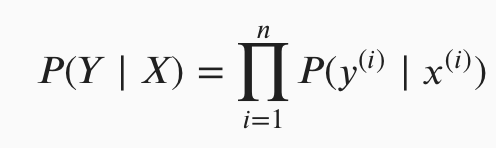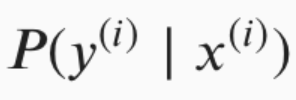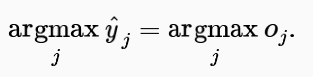# Softmax Regression

Sorry, I can’t recognize the math formulas in 3.4.6.The first part is the definition of the joint likelihood function:How likely it is that we observe the classifications that we do, conditional on the the input data we have and the parameters we choose (the full version of this should include the parameters after x in some form I think). We’re trying to maximise this: to choose the parameters that make the combination of input data & actual labels that we observed as likely as possible.

The second part is just a mathematical manipulation of the first part:

<image of the second part that I can’t insert because I’m new)

So we can note that maximising the first is the same as minimising the second (since we took the negative of it), and therefore we can treat the second as a ‘loss function’ (thing we want to minimise).

Thanks. I already have known what -log does. But I still wonder the math meaning and, more importantly , realistic meaning of the definition of the joint likelihood function.

Why would we need to multiply all P(y|x)?
Do their parameters independent surely?

If we multiply some numbers which stands for different meanings, the meaning of the result is hard to say.

And I think probability is often illusive if we only use pure math without any other descripition of a natural language.

Hi @StevenJokes,
$P(Y | X) = P(y_1, y_2, … | x_1,x_2,…) = \prod{P(y_i | x_i)}$ since the samples are independent.

Are we sure about the independence? How can we? Or we just naively think that all samples are independent?
I think that independence and the establish of $P(Y | X) = P(y_1, y_2, … | x_1,x_2,…) = \prod{P(y_i | x_i)}$ 's equal sign are mutual causation.In other words, just logical rename.

Thanks. But I think I’m still confused. Are we sure about the independence? How can we? Or we just naively think that all samples are independent?
I think that independence and the establish of $P(Y | X) = P(y_1, y_2, … | x_1,x_2,…) = \prod{P(y_i | x_i)}$ 's equal sign are mutual causation. In other words, just logical rename. We should know what P*P refers in the real world.And I think probability just refers to how much fraction of some real whole thing, but we use it abusely to try to predict, such as whether head or tail a coin will face up nextly.
And it is understandable to use it to process natural language due to consistency required in Logic.
But we still need more information to predict a dynamic process. And we just want to be a peacemaker when we say that P = 1/2, then we will be confident to not be wrong too much.

And we can’t predict any if we can’t describe what will happen and what situation we are in now.
And different ways to describe a thing usually confuses each other, then another story will happen.
The attempts to simplify our world by concluding everything maybe just deduce a contrary result that the world will be more complex due to typo, syntax error, lie, misunderstanding, distrust and so on.Just my random thoughts.I think we do assume that the samples are conditionally independent yes - after conditioning on the input data x_i, the y_i values are independent of each other. I think this comes from assuming that the Gaussian noise in the model is well-behaved (iid).

The book has more to say on this in section 4.4.1.1:

In the standard supervised learning setting, which we have addressed up until now and will stick with throughout most of this book, we assume that both the training data and the test data are drawn independently from identical distributions (commonly called the i.i.d. assumption). This means that the process that samples our data has no memory.

1 Like

No memory is too abstract. What does it mean?
I need more examples of identical distrbutions.
What can we call that they are identical distributions?
For example, students in a class are identical distributions?
But they usually have similiar ages and live near the school.

I read an example of it:

Just like if I flip the dice and I flip it every time, that’s independent. But if I want the sum of the two flips to be greater than 8, and the rest doesn’t count, then the first flip and the second flip are not independent, because the second flip is dependent on the first flip

What I want globally will change the dependence of data itself. Am I right?
If so, I can say it is dependent or not, just by my thought.

And I think the probablity is just an ideal thought by some mathematicians who has crazy thought to predict everything just by math.

“No memory” means we don’t use the result from the first trail to judge the second trail result (positive or negative). That is, the second trail outputs fresh new positive or negative result, it doesn’t depend on the first one.

So, we only wait for the result of the second to happen?
Probability, in the begining, more depends on what we think how many categories result are.
And, because we have freedom to say, inconsistency is so common, such as famous “Bayesian method”.
Bayesian method actually looks like a good way that we try to put right after finding something wrong.
But it is hard to say that, what we tried to fix in past will be still effective in the future.Again, my random thought!

If we can fully use the past experience to guide our action in future, then how similar of past and future is the next question that we should take attention to.
In my understanding,
over-fitting problems focus more on difference of past and future, while
under-fitting on similarity.

In exercise 1:

• Compute the second derivative of the cross-entropy loss 𝑙(𝐲,𝐲̂) for the softmax.

Is that means

?
And where can I find exercises answer?

Hi @sheey, the second derivative will be:

$\frac{\partial^2 l(\mathbf{y}, \hat{\mathbf{y}})}{{{o_j^2}}} = … = \mathrm{softmax}(\mathbf{o})_j \cdot (1- \mathrm{softmax}(\mathbf{o})_j)$

i.e.,

Sorry we currently don’t provide the solutions. But feel free to ask question at the discussion forum1 Like

j should be in the bracket?

or
When o is vector,j should be outside?
When o_j,j should be in?We only need to calculate the the derivative of softmax(o)_j （j is in or out?) to get the second derivative of the cross-entropy loss 𝑙(𝐲,𝐲̂) ?
I noticed that the second derivative of the cross-entropy loss 𝑙(𝐲,𝐲̂) is exactly the derivative of softmax(o)_j .
The derivative of y_j is 0 ? y_j is 1 or 0? j represents the label?

Hi @StevenJokes, good question! Actually, $j$ should be outside, since we first calculate softmax of the vector $o$, then take its j’s component.

Yes. I don’t fully understand your question though.

I got it. Thanks @goldpiggy